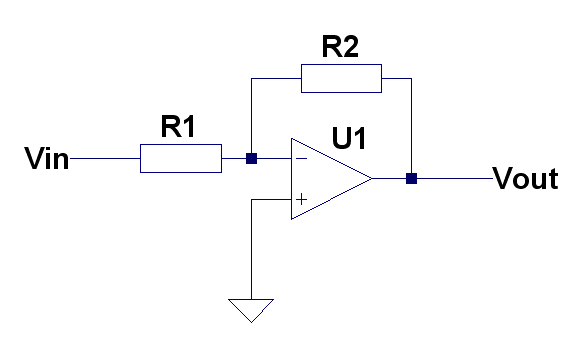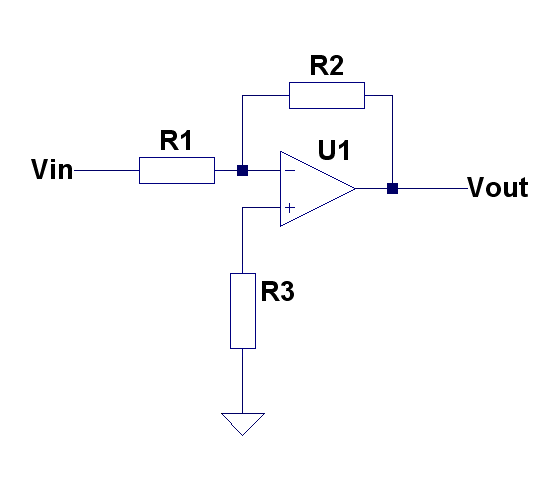# Inverting amplifierVout = -(R2/R1)*Vin

or

Gain = -R2/R1

If taking input offset voltage and currents into account:

Vout = -(R2/R1)*(Vin + Vofs + R2/Iin-).

## Canceling effect of input bias currentR3=R1

Vout = -(R2/R1)*(Vin + R2/Iofs-)

• The effect of input offset voltage/bias current is amplified by the gain. For example if the opamp has 3mV offset voltage and gain of 100, then the absolute output error due offset voltage will be 0.3V.
• R3 is optional and should be equal to R1. It cancels effect of input current. The highter R1 is, the higher will be error due input bias current (if R3 is missing). The input offset current (difference between current on positive and negative inputs) still affects the output. Use lower values for R1 and R3 if the input offset current error is too big.